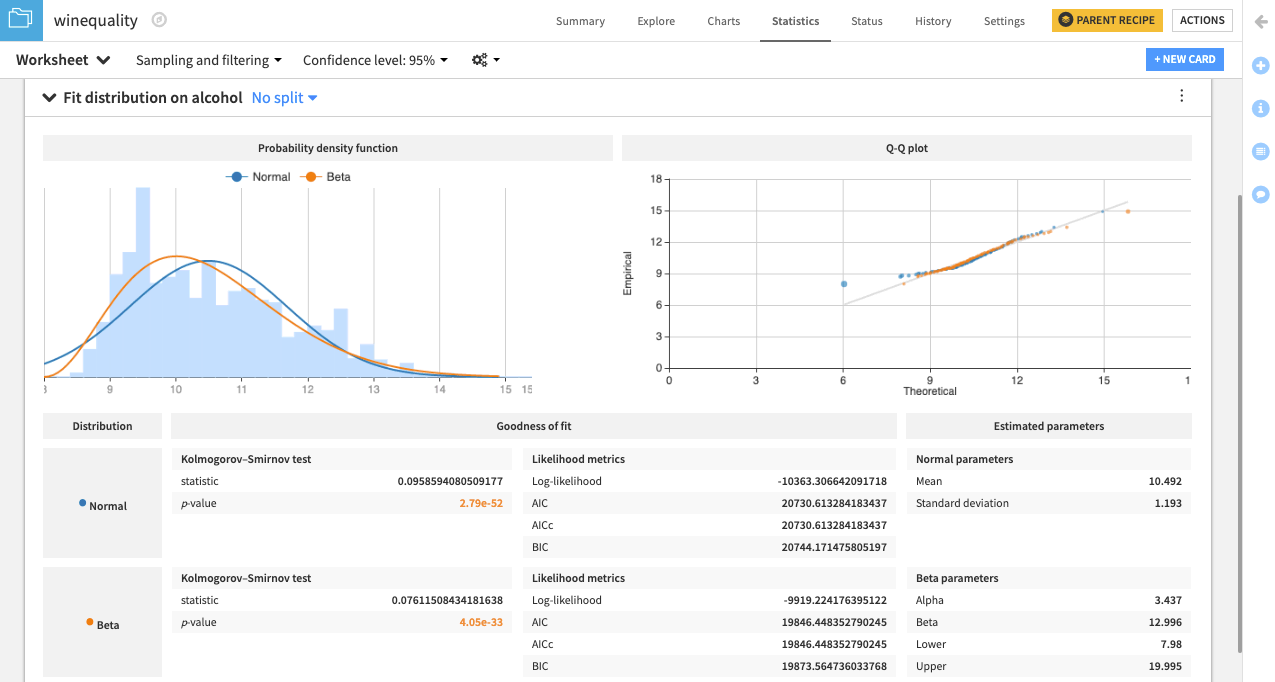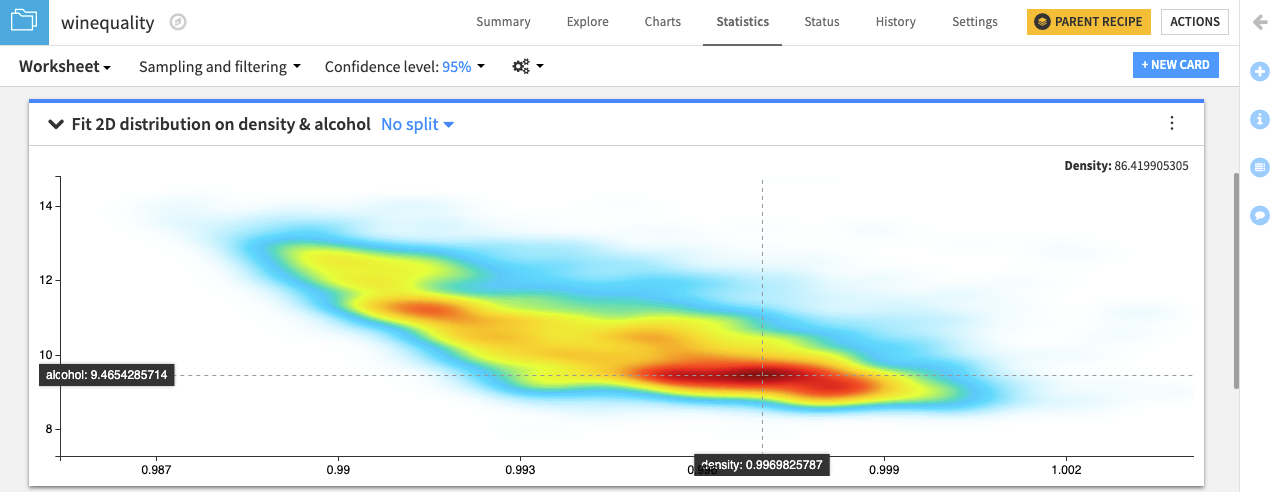# Tutorial | Fit univariate and bivariate distributions¶

Note

This lesson is a continuation of the Interactive Statistics hands-on tutorial.

## Univariate distributions¶

Another aspect of descriptive statistics involves modeling the probability distribution of your dataset.

Dataiku allows you to estimate the parameters of univariate probability distributions using the Fit Distribution card. This feature is available only for numerical variables.

Let’s attempt to fit the Normal and Beta distributions to the dataset, considering only the alcohol variable.

• Click the New Card button from the Worksheet header and choose Fit curves & distributions.

• Select the Fit Distribution card.

• Select alcohol as the Variable and Normal as the Distribution.

• Add another distribution by clicking the +Add a Distribution box and selecting Beta.

• Click Create Card.Dataiku creates a card that shows the normal and beta probability density functions fit to the data. There is also a Q-Q plot that compares the quantiles of the data to the quantiles of the fitted distributions. Observing points that are far from the identity line suggests that the data could not have been drawn from either distribution.

Additionally, the card includes goodness of fit metrics and the estimated parameters for the normal and beta distributions.

## Bivariate distributions¶

Similarly, the 2D Fit Distributions card is available for visualizing and estimating bivariate probability distributions on your dataset.

Let’s attempt to fit a 2D kernel density estimate (KDE) to the dataset, considering only the density and alcohol variables.

• Click the New Card button from the Worksheet header and choose Fit curves & distributions.

• Select the 2D Fit Distribution card.

• Specify density as the X Variable and alcohol as the Y Variable.

• Select the 2D KDE radio button. Notice that the X relative bandwidth and Y relative bandwidth have the default value of 15. Let’s keep these default values. However, you can increase the values to make the KDE plot smoother, or decrease the values to make the plot less smooth.

• Click Create Card to create the card.Note

For more information, see Fit curves and distributions in the reference documentation.